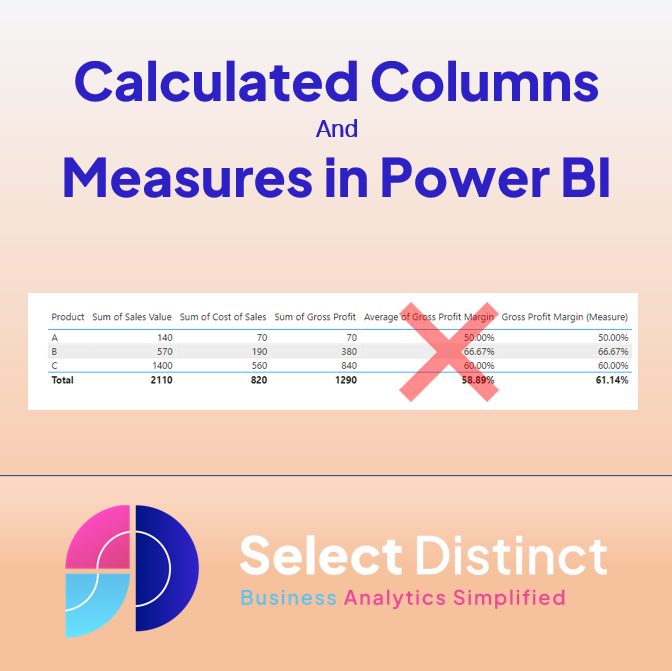## Calculated Columns and Measures in Power BIIntroduction to Calculated Columns and Measures in Power BI A guide to understanding the differences between calculated columns and measures in DAX for Power BI   What is a calculated column? A calculated column is a static value added to a table, calculated row by row when you load the data. What is a measure? […]